## Not applicable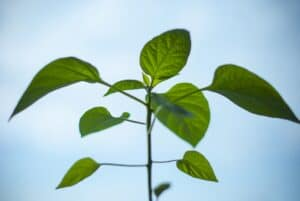In this activity students conduct a video measurement of the growth of the root and stem of a plant after germination. They analyse the growth curve of the plant and couple the growth speed to available energy.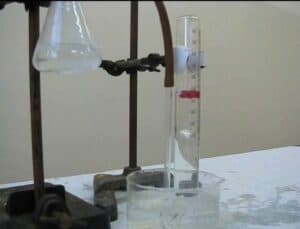In this activity students study the reaction between magnesium and hydrochloric acid using a pre-recorded video. The reaction equation shows that a gas is produced. By measuring the amount of gas that is produced during a certain amount of time, the reaction rate can be determined. Students follow the rate of gas production by following the position of floating plastic balls in the collecting cylinder. By using the correct scaling, the moment of the balls can be correlated to a certain volume of gas. This scaling has already been prepared so that students can focus on the chemical aspects of this experiment.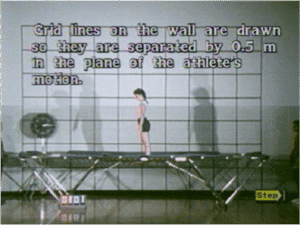In this activity students measure on the video clip and graph the movement of a trampoline jumper. Students create the velocity and acceleration versus time graphs and analyse both graphs. They investigate the relationship investigate the relationship between the position, the velocity and the acceleration of the jumper.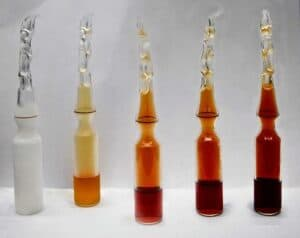In this activity students study an existing model of an irreversible reaction of N2O4 to NO2 and expand it in order to create a chemical equilibrium. In order to teach students to critically assess a model, the concentration quotient has to be calculated at some point in the activity. This activity focusses on the essence of modelling and less on the workings of an equilibrium.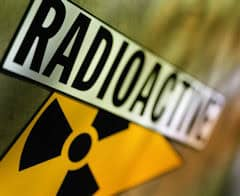In this activity students explore radioactive decay via a given decay model. Based on the model they determine the function, which describes the radioactive decay. Further they modify the model to describe a decay of a real radioactive isotope e.g. Barium 137m and compare how well the model fits the experimental results.